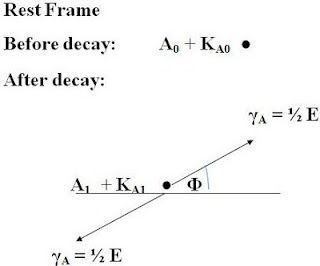## Saturday, June 01, 2013

### Einstein's Derivation of the Famous Equation, E=mc2

Here’s a quick rundown on one of the most famous equation in physics, E = mc2. Einstein knew from experiments previously done that a particle could decay and release gamma rays. He reasoned that when this happened, the particle would lose kinetic energy, and this could only be accounted by a loss of mass. So how did he come to that conclusion? He analysed the situation both in a rest frame and in a moving frame.The value of γ was already known, but we can derive it from Relativistic Doppler Effect (to be the topic for another blog A).

By the law of conservation of energy:

The energy before decay = the energy after decay

(1) In the rest frame:

A0 + KA0 = A1 + KA1 + ½ E + ½E = A1 + KA1 + E

(2) In the moving frame:
B0 + KB0 = B1 + KB1 + ½ E(1 – (v/c)cosΦ)(1 – v2/c2)
+ ½ E(1 + (v/c)cosΦ)(1 – v2/c2)

= B1 + KB1 + E(1 – v2 /c2)

Now taking a look at the energy difference of the particle in each of the frame:

(3) In the rest frame:

A1 – A0 = KA0 – E – KA1 = – ΔKA – E

(4) In the moving frame:

B1 – B0 = KB0 – KB1 – E(1 – v2 /c2)
= – ΔKB – E(1 – v2 /c2)

Whether the observer is at rest or moving with respect to the particle, the energy difference should be the same.

(5) – ΔKA – E = – ΔKB – E(1 – v2 /c2)

Calculating the difference in kinetic energy:

((6) ΔK = ΔKA – ΔKB = E(1 – v2 /c2) – E

= E ((1 – v2/c2) – 1)

≈ E ((1 + ½v2/c2) – 1)

= ½ E (v2/c2)

Einstein had reasoned that if the kinetic energy of the particle is smaller by ½ E (v2/c2), the only way this can happen is that the particle must lose mass when emitting radiation.

By definition the kinetic energy is,

(7) K = ½ mv2

If the particle was initially at rest,

(8) ΔK = K = ½ mv2

Equating (6) and (8)

½ mv2 = ½ E (v2/c2)

(9) Therefore E = mc2.